# Runge-Kutta method

A one-step method for numerically solving the Cauchy problem for a system of ordinary differential equations of the form(1)

The principal idea of the Runge–Kutta method was proposed by C. Runge  and developed later by W. Kutta  and others. Originally, this idea was used only for constructing explicit schemes of the method, which were sought in the form(2)

where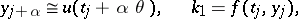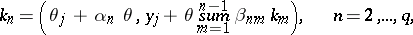and, moreover, the values of the constants,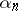,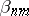,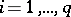;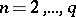;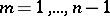, were defined from the requirement that the error of equation (2) on the exact solution of (1) should have the highest order (i.e. power of, the stepsize, in the leading term of the expansion).

In contrast to multi-step methods, the Runge–Kutta method, as other one-step methods, only requires the value at the last time point of the approximate solution and allows one to carry out calculations under initial conditions which are natural for equation (1). This allows one to use it directly also for not equally-spaced grids. However, since this method does not make use of information concerning the solution at previous nodes of the grid, it is, in general, less economical than, e.g., the Adams method.

The classical Runge–Kutta method (see, e.g., ) is the method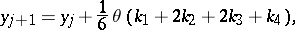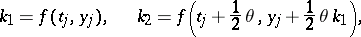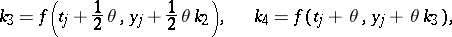which belongs to the family of methods with fourth order of accuracy of the form (2) with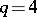, depending on two free parameters. The simplest explicit Runge–Kutta with first order of accuracy is obtained from (2) when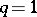; it is also the most widely used. This method is known as Euler's method. From (2) for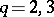one can obtain families of Runge–Kutta methods with second and third order of accuracy depending on one and two free parameters, respectively. For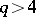the above correspondence between the value ofand the optimal order of accuracy is no more valid. Runge–Kutta methods of the form (2) with fifth order of accuracy can be realized only for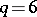, sixth order — for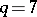, seventh order — for, etc. In this case, if one increasesby one, extension of the set of constants,,to be chosen in (2) is often insufficient to satisfy the conditions resulting from the requirement to increase the order of accuracy of an explicit Runge–Kutta method by one. To increase the number of parameters to be chosen in (2) one can consider, for example, the following extension of the construction of one-step methods based on the concept of Runge: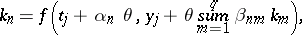(3)In general, methods of the form (2), (3) are already implicit. This complicates significantly their numerical implementation: The values,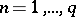, at each step have to be found from the system of, generally speaking, non-linear equations (3). However, due to a considerable increase in the number of constants to be chosen, such methods acquire the following property (see ): For any value ofthere exists an implicit Runge–Kutta method of order of accuracy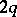. Moreover, under such extension of the class of Runge–Kutta methods there arise methods well adapted to stiff differential systems (cf. Stiff differential system).

There exists one more modification (see, e.g., ) of Runge's concept of constructing one-step methods for numerically solving equations of the form (1). Namely, proceeding from (1) one writesAn approximation of the latter integral by a quadrature formula withnodes gives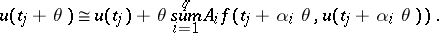(4)

If the choice of nodes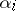and coefficients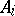,, of the considered quadrature formula is submitted to the conditions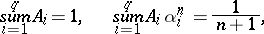(5)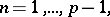then the error of the approximate equality (4) will be of order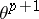. Forthe system of equations (5) is solvable and the approximate equality (4) can be constructed. Similarly, one can write approximate equalities for the unknown values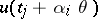in the right-hand side of (4); moreover, restrictions on their accuracy may be in lowering the order, etc.

An example of a one-step method constructed in the way mentioned above is the following method with third order of accuracy, of predictor-corrector type (see ):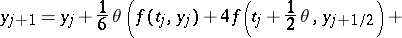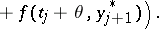If one assumes that in (4) one ofis, then in the same way one can also construct implicit methods, e.g., the method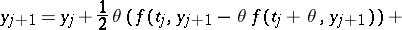with second order of accuracy.

The approaches to the construction of numerical methods considered above for equations of type (1) can be extended to ordinary differential equations of higher orders (see , ) and are also used when constructing difference schemes in the case of partial differential equations.

How to Cite This Entry:
Runge-Kutta method. Encyclopedia of Mathematics. URL: http://encyclopediaofmath.org/index.php?title=Runge-Kutta_method&oldid=22999
This article was adapted from an original article by V.V. Bobkov (originator), which appeared in Encyclopedia of Mathematics - ISBN 1402006098. See original article